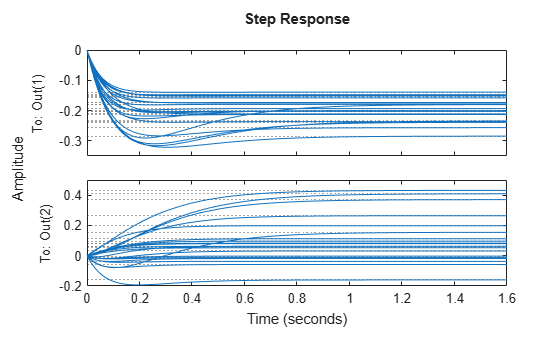# Sample Uncertain Systems

The command `usample` randomly samples the uncertain system at a specified number of points. Randomly sample an uncertain system at 20 points in its modeled uncertainty range. This gives a 20-by-1 `ss` array. Consequently, all analysis tools from Control System Toolbox™ are available.

```p1 = ureal('p1',10,'Percentage',50); p2 = ureal('p2',3,'PlusMinus',[-.5 1.2]); p3 = ureal('p3',0); A = [-p1 p2; 0 -p1]; B = [-p2; p2+p3]; C = [1 0; 1 1-p3]; D = [0; 0]; sys = ss(A,B,C,D) % Create uncertain state-space model```
```sys = Uncertain continuous-time state-space model with 2 outputs, 1 inputs, 2 states. The model uncertainty consists of the following blocks: p1: Uncertain real, nominal = 10, variability = [-50,50]%, 2 occurrences p2: Uncertain real, nominal = 3, variability = [-0.5,1.2], 2 occurrences p3: Uncertain real, nominal = 0, variability = [-1,1], 2 occurrences Type "sys.NominalValue" to see the nominal value, "get(sys)" to see all properties, and "sys.Uncertainty" to interact with the uncertain elements. ```
```manysys = usample(sys,20); size(manysys)```
```20x1 array of state-space models. Each model has 2 outputs, 1 inputs, and 2 states. ```
`stepplot(manysys)`The command `stepplot` can be called directly on a `uss` object. The default behavior samples the `uss` object at 20 instances, and plots the step responses of these 20 models, as well as the nominal value.

The same features are available for other analysis commands such as `bodeplot`, `bodemag`, `impulse`, and `nyquist`.